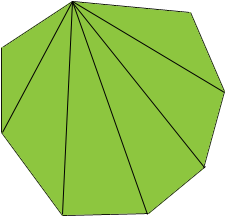How do you figure the area of an irregular octagon? Measurements are 4 equal sides of =BD", and 4 equal sides of =BE". It measures 1 1/2" = from flat to flat. Thank you, Billy Billy, One technique is to subdivide the octagon into six triangles as in the diagram.If you can measure the sides of the six triangles then you can find their areas using Heron's formula. The area of the octagon is the sum of the areas of the six triangles. It may be possible to find the area of your octagon with fewer calculations but without seeing the actual shape of the octagon the technique above is the best I can do. Penny